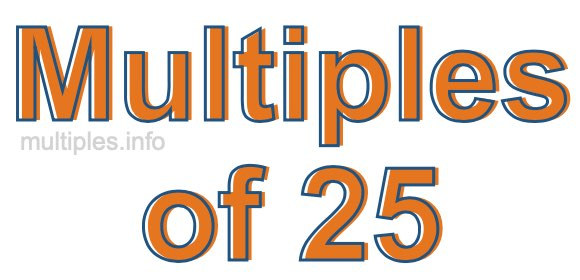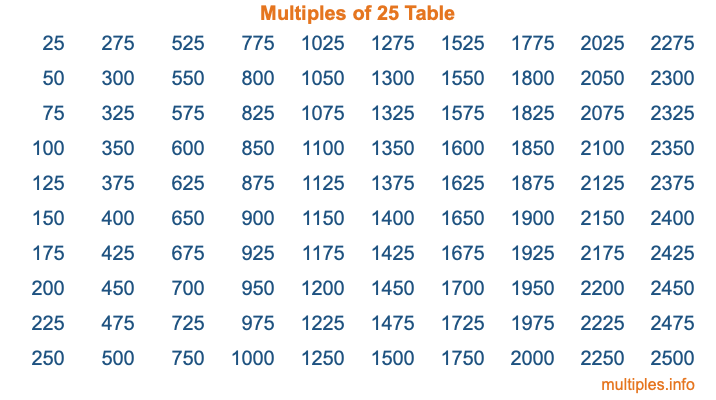Multiples of 25Welcome to the Multiples of 25 page. Here we will first teach you everything you will ever need to know about the multiples of 25, and then give you a study guide summary of everything we taught you to make sure you remember it all. Use this page to look up facts and learn information about the multiples of 25. This page will make you a multiples of twenty-five expert!

Definition of Multiples of 25
Multiples of 25 are all the numbers that when divided by 25 equal an integer. Each of the multiples of 25 are called a multiple. A multiple of 25 is created by multiplying 25 by an integer.

Therefore, to create a list of multiples of 25, you start with 1 multiplied by 25, then 2 multiplied by 25, then 3 multiplied by 25, and so on for as long as you want. Thus, the list of the first five multiples of 25 is 25, 50, 75, 100, and 125. To see a larger list of multiples of 25, see the printable image of Multiples of 25 further down on this page. We also have a category where you can choose any nth multiple of 25.

Multiples of 25 Checker
The Multiples of 25 Checker below checks to see if any number of your choice is a multiple of 25. In other words, it checks to see if there is any number (integer) that when multiplied by 25 will equal your number. To do that, we divide your number by 25. If the the quotient is an integer, then your number is a multiple of 25.

Is  a multiple of 25?

Least Common Multiple of 25 and ...
A Least Common Multiple (LCM) is the lowest multiple that two or more numbers have in common. This is also called the smallest common multiple or lowest common multiple and is useful to know when you are adding our subtracting fractions. Enter one or more numbers below (25 is already entered) to find the LCM.

Check out our LCM Calculator if you need more details about the Least Common Multiple or if you need the LCM for different numbers for adding and subtraction fractions.

nth Multiple of 25
As we stated above, 25 is the first multiple of 25, 50 is the second multiple of 25, 75 is the third multiple of 25, and so on. Enter a number below to find the nth multiple of 25.

th multiple of 25

Multiples of 25 vs Factors of 25
25 is a multiple of 25 and a factor of 25, but that is where the similarities end. All postive multiples of 25 are 25 or greater than 25. All positive factors of 25 are 25 or less than 25.

Below is the beginning list of multiples of 25 and the factors of 25 so you can compare:

Multiples of 25: 25, 50, 75, 100, 125, etc.

Factors of 25: 1, 5, 25

As you can see, the multiples of 25 are all the numbers that you can divide by 25 to get a whole number. The factors of 25, on the other hand, are all the whole numbers that you can multiply by another whole number to get 25.

It's also interesting to note that if a number (x) is a factor of 25, then 25 will also be a multiple of that number (x).

Multiples of 25 vs Divisors of 25
The divisors of 25 are all the integers that 25 can be divided by evenly. Below is a list of the divisors of 25.

Divisors of 25: 1, 5, 25

The interesting thing to note here is that if you take any multiple of 25 and divide it by a divisor of 25, you will see that the quotient is an integer.

Multiples of 25 Table
Below is an image of the first 100 multiples of 25 in a table. The table is in chronological order, column by column. The first column has the first ten multiples of 25, the second column has the next ten multiples of 25, and so on.The Multiples of 25 Table is also referred to as the 25 Times Table or Times Table of 25. You are welcome to print out our table for your studies.

Negative Multiples of 25
Although not often discussed or needed in math, it is worth mentioning that you can make a list of negative multiples of 25 by multiplying 25 by -1, then by -2, then by -3, and so on, to get the following list of negative multiples of 25:

-25, -50, -75, -100, -125, etc.

Multiples of 25 Summary
Below is a summary of important Multiples of 25 facts that we have discussed on this page. To retain the knowledge on this page, we recommend that you read through the summary and explain to yourself or a study partner why they hold true.

There are an infinite number of multiples of 25.

A multiple of 25 divided by 25 will equal a whole number.

25 divided by a factor of 25 equals a divisor of 25.

The nth multiple of 25 is n times 25.

The largest factor of 25 is equal to the first positive multiple of 25.

25 is a multiple of every factor of 25.

25 is a multiple of 25.

A multiple of 25 divided by a divisor of 25 equals an integer.

25 divided by a divisor of 25 equals a factor of 25.

Any integer times 25 will equal a multiple of 25.

Multiples of a Number
Here you can get the multiples of another number, all with the same attention to detail as we did for multiples of 25 on this page.

Multiples of
Multiples of 26
Did you find our page about multiples of twenty-five educational? Do you want more knowledge? Check out the multiples of the next number on our list!